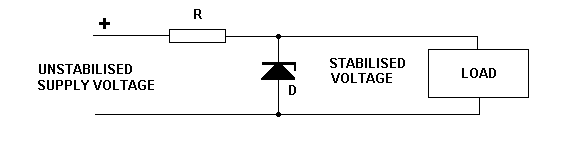Electronic Circuits and Tutorials
Tutorials
Dictionaries
Projects
Discover
Home > Electronics Tutorial > The Diode Tutorial > Zener Diode Tutorial
The Diode - Electronic Tutorials

# Zener Diode TutorialThe Zener diode is operated in reverse bias mode (positive on its cathode). It relies on the reverse breakdown voltage occurring at a specified value. This value is printed on it. It has two main applications.

1. as a reference source, where the voltage across it is compared with another voltage.

2. as a voltage regulator, smoothing out any voltages variations occurring in the supply voltage across the load.

When being used a voltage regulator, if the voltage across the load tries to rise then the Zener takes more current. The increase in current through the resistor causes an increase in voltage dropped across the resistor. This increase in voltage across the resistor causes the voltage across the load to remain at its correct value.

In a similar manner, if the voltage across the load tries to fall, then the Zener takes less current. The current through the resistor and the voltage across the resistor both fall. The voltage across the load remains at its correct value.

Note: To report broken links or to submit your projects, tutorials please email to Webmaster